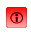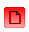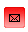Exposing the personalitybehind the pen... Friday, June 9, 2023News Release

 Medical study preliminary results indicate five of the six writematch traits measured show positive relationship June 28, 2003    by:   WriteMatch.com The following writematch traits were all independently evaluated against the (MS) Diagnosis Category: Rhythm Legibility Stroke Quality Tremor Irregularity Form Based on the "ordinal data" that was being measured, the Spearman Rank-Order Correlation Coefficient was determined for a bivariate set of paired XY rankings. The results were as follows: MS Diagnosis Category (x-axis) / Rhythm (y-axis): Correlation Coefficient = -0.0431 MS Diagnosis Category (x-axis) / Legibility (y-axis): Correlation Coefficient = 0.0922 MS Diagnosis Category (x-axis) / Stroke Quality (y-axis): Correlation Coefficient = 0.1669 MS Diagnosis Category (x-axis) / Tremor (y-axis): Correlation Coefficient = 0.1302 MS Diagnosis Category (x-axis) / Irregularity (y-axis): Correlation Coefficient = 0.0963 MS Diagnosis Category (x-axis) / Form (y-axis): Correlation Coefficient = 0.1827 Due to the relatively small same size of n=54, the five of the six independent studies that proved a positive correlation were not significant. If the trends that were found in this study continue and the aggregated subject size expands from 54 samples (for all the studies except the "Stroke Quality" which only had 35 valid samples) to between 100 and 150 samples, two to three of the six writematch physiological aspects that were measured would prove to show a significant linear relationship with the change in MS Diagnosis Category. If a significant relationship can be established for any of these writematch characteristics, the next step would be focused on a predictive study such as Logistic Regression.